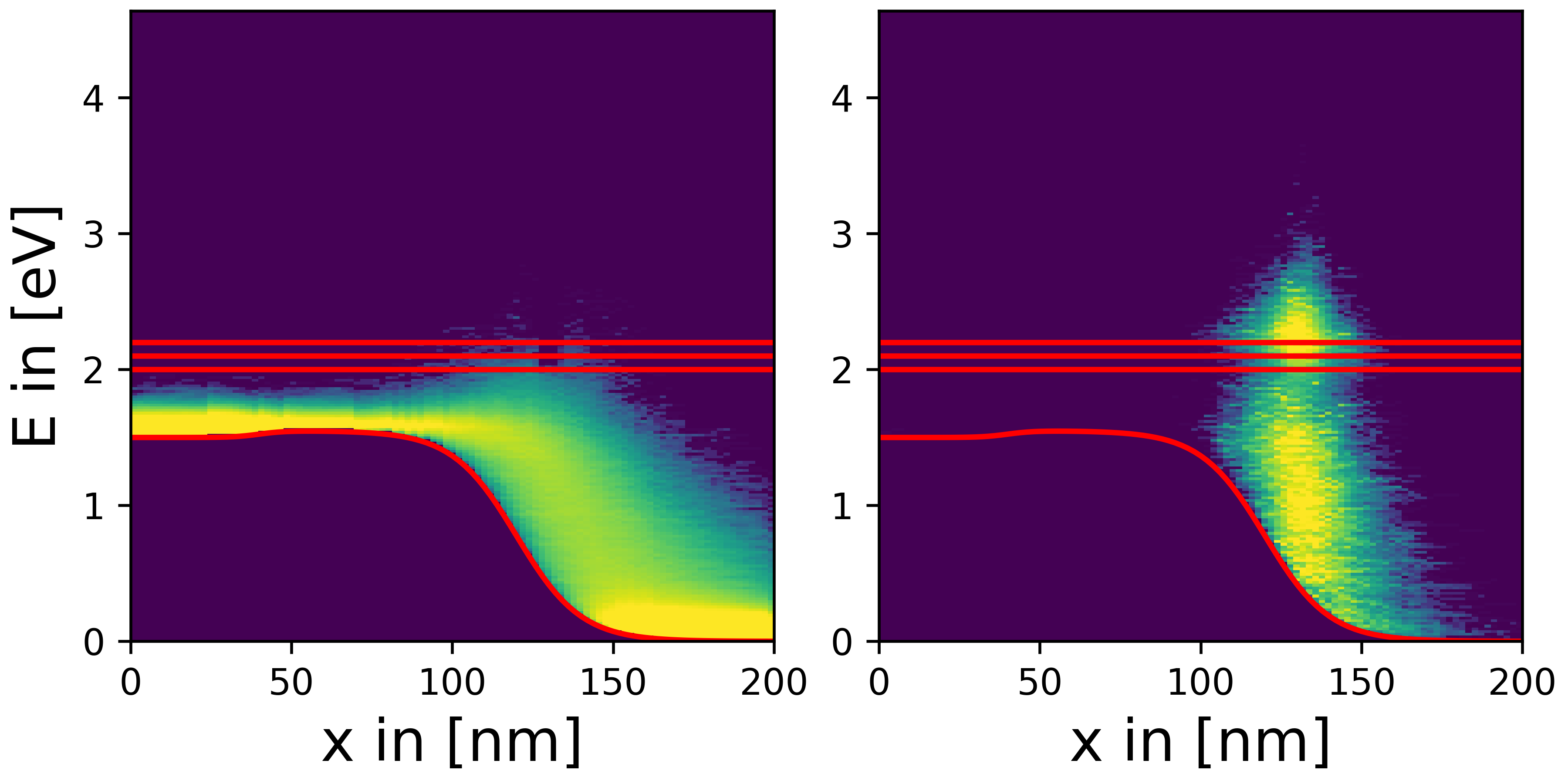Hans Kosina Ao.Univ.Prof. Dipl.-Ing. Dr.techn. Publications### Biography

Hans Kosina received the Diplomingenieur degree in electrical engineering and PhD from the Technische Universität Wien in 1987 and 1992, respectively. He was with the Institute of Flexible Automation at the Technische Universität Wien for one year and then joined the Institute for Microelectronics, where he is currently an associate professor. He received the venia docendi in microelectronics in 1998. In the summer of 1993, he was a visiting scientist at Motorola Inc., Austin, Texas, and in the summer of 1999, a visiting scientist at Intel Corp., Santa Clara, California. Dr. Kosina was chairman of the ''11th International Workshop on Computational Electronics'' held in Vienna in May 2006 and is a member of the advisory board of the International Workshop on Computational Nanotechnology. He has served as an Associate Editor of the IEEE Transactions on Computer-Aided Design of Circuits and Systems and the Journal of Computational Electronics. His current research interests include nanoelectronic device modeling and the development of numerical methods and algorithms for classical and quantum transport calculations.

## Numerical Methods in Quantum Transport

Modeling of an open quantum system requires knowledge of the surface Green’s function (GF). This quantity is usually calculated using the iterative method proposed by Sancho and Rubio in 1985. It has several problems, however. Since a matrix inversion is required at each iteration step, this method can result in time-consuming calculations. Artificial damping has to be introduced to achieve convergence, which can also affect the accuracy of the results. Over the last year, we have derived a spectral method that allows a direct evaluation of the surface GF. The latter represents the impulse response of a linear system, which can be readily stated in reciprocal space. The inverse Fourier transform yields the impulse response in configuration space. For small Hamiltonian matrices, one can obtain analytical expressions for the surface GF. We have considered the Dirac-like Hamiltonian of graphene and the k*p Hamiltonian of 1T' molybdenum disulphide which is a topological insulator.

A new project on numerical methods for the stationary Wigner and the von Neumann equation has been started. The Wigner equation is often solved using a discretization scheme proposed by William Frensley three decades ago. The results obtained using this scheme have been shown to strongly depend on the discretization parameters, especially the mesh spacing. The stationary Wigner equation has a singularity at zero momentum (k = 0). Our understanding is that the breakdown of Frensley's method is due to the improper treatment of that singularity. In this project, we are investigating a new discretization scheme that explicitly includes the point k = 0. The resulting equation system is over-determined since one obtains two equations for that point. The first one is an algebraic constraint that ensures the regularity of the solution at k = 0. The second equation is the transport equation evaluated at k = 0. The resulting system, which we refer to as the constrained Wigner equation, is over-determined and can only be solved approximately. The main goal of the project is the development of such approximate solution methods. We will first systematically study both the constrained Wigner equation and the von Neumann equation in a single spatial dimension and develop numerical solution methods. In a second step, we will explore the extension of the method to two spatial dimensions and parallelize the numerical algorithms developed.

An ongoing project on negative capacitance and ferroelectric transistors has entered its second year. The primary focus of the work done over the past year has been to develop physical and computer models that are capable of modeling both charge trapping and ferroelectric switching simultaneously. These models are validated against experimental data, and a current goal is to develop a potential experimental protocol for characterizing the strength and nature of these effects present in a given real device, as well as to suggest new designs to combat the issue. The trapping physics is currently being handled by Comphy, a pre-existing code-base within the Institute, with the ferroelectric physics being handled by novel kinetic Monte Carlo models.Fig. 1: Current-voltage characteristics of a resonant tunneling diode computed by different methods. Results of the constrained von Neumann equation (sigma) and the Schroedinger equation (QTBM) show good agreement. The effect of grid refinement on the von Neumann result is also shown.